# Making Data Normal Using Box-Cox Power Transformation

By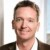Arne Buthmann

Normally distributed data is needed to use a number of statistical analysis tools, such as individuals control charts, Cp/Cpk analysis, t-tests and analysis of variance (ANOVA). When data is not normally distributed, the cause for non-normality should be determined and appropriate remedial actions should be taken. (An introduction to remedial actions for non-normal data can be found in “Dealing with Non-normal Data: Strategies and Tools.”)

Data transformation, and particularly the Box-Cox power transformation, is one of these remedial actions that may help to make data normal. By understanding both the concept of transformation and the Box-Cox method, practitioners will be better prepared to work with non-normal data.

### What Are Transformations?

Transforming data means performing the same mathematical operation on each piece of original data. Some transformation examples from daily life are currency exchange rates (e.g., U.S. dollar into Euros) and converting degree Celsius into degree Fahrenheit.

These two transformations are called linear transformations because the original data is simply multiplied or divided by a specific coefficient or a constant is subtracted or added. But these linear transformations do not change the shape of the data distribution and, therefore, do not help to make data look more normal (Figure 1).

### What is the Box-Cox Power Transformation?

The statisticians George Box and David Cox developed a procedure to identify an appropriate exponent (Lambda = l) to use to transform data into a “normal shape.” The Lambda value indicates the power to which all data should be raised. In order to do this, the Box-Cox power transformation searches from Lambda = -5 to Lamba = +5 until the best value is found. Table 1 shows some common Box-Cox transformations, where Yis the transformation of the original data Y. Note that for Lambda = 0, the transformation is NOT Y (because this would be 1 for every value) but instead the logarithm of Y.

 Table 1: Common Box-Cox Transformations l Y’ -2 Y-2 = 1/Y2 -1 Y-1 = 1/Y1 -0.5 Y-0.5 = 1/(Sqrt(Y)) log(Y) 0.5 Y0.5 = Sqrt(Y) 1 Y1 = Y 2 Y2

An example: Figure 2 shows non-normally distributed cycle time data. Using the Box-Cox power transformation in a statistical analysis software program provides an output that indicates the best Lambda values (Figure 3).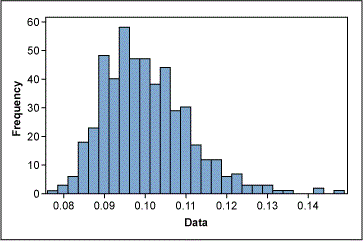Figure 2: Example of Non-normally Distributed Cycle Time Data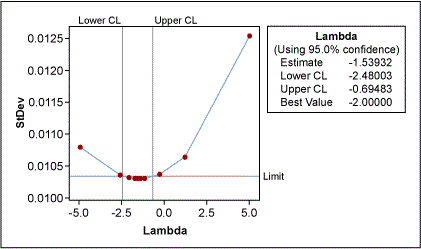Figure 3: Example Box-Cox Plot of Data

The lower and upper confidence levels (CLs) show that the best results for normality were reached with Lambda values between -2.48 and -0.69. Although the best value is -1.54 (estimate in Figure 3), the process works better if this value is rounded to a whole number; this will make it easier to transform the data back and forth. The best whole-number values here are -1 and -2 (the inverse function of Y and Y2, respectively). The histogram in Figure 4 shows the transformed data using Lambda = -1, now more normally distributed.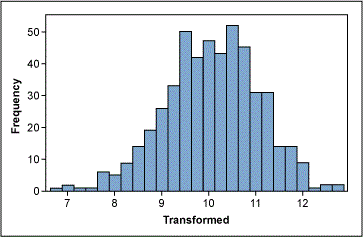Figure 4: Data Transformed Using Lambda = -1

### Does Box-Cox Always Work?

The Box-Cox power transformation is not a guarantee for normality. This is because it actually does not really check for normality; the method checks for the smallest standard deviation. The assumption is that among all transformations with Lambda values between -5 and +5, transformed data has the highest likelihood – but not a guarantee – to be normally distributed when standard deviation is the smallest. Therefore, it is absolutely necessary to always check the transformed data for normality using a probability plot.

Handpicked Content:   Dealing with Non-normal Data: Strategies and Tools

Additionally, the Box-Cox Power transformation only works if all the data is positive and greater than 0. This, however, can usually be achieved easily by adding a constant (c) to all data such that it all becomes positive before it is transformed. The transformation equation is then:

Y’ = (Y+C)l

### Application Example

A project team collected cycle time data from a purchase order-generation process. One team member created a control chart of this data (Figure 5) and was about to ask what special cause had happened for data point 40 when the Green Belt remembered that using an individuals control chart requires normally distributed data. A look at the probability plot of the data (Figure 6) revealed non-normal distribution. Therefore, the control limits of the control chart were useless.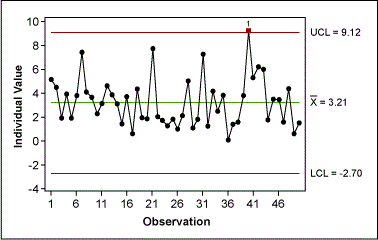Figure 5: Control Chart of Original Cycle Time Data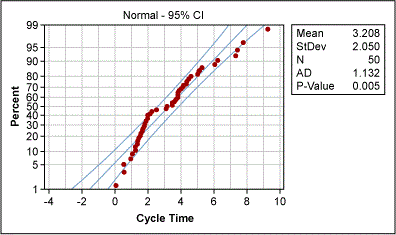Figure 6: Probability Plot of Original Cycle Time Data

The Green Belt used the Box-Cox power transformation to determine whether the data could be transformed (Figure 7). Box-Cox suggested a best Lambda value of 0.5 for transformation (i.e., the square root of the original data). And the transformation really worked: The new probability plot confirms normality (Figure 8).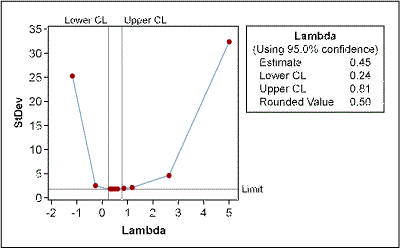Figure 7: Box-Cox Plot of Cycle Time Data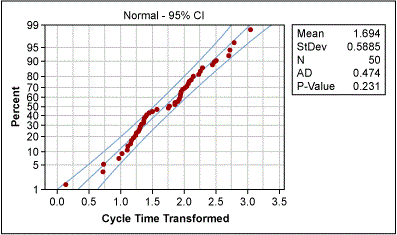Figure 8: Probability Plot of Transformed Cycle Time Data

After the transformation, the Green Belt created a control chart of the transformed data and showed that the purchase order-generation process was actually quite stable, i.e., all variation was due to common causes (Figure 9).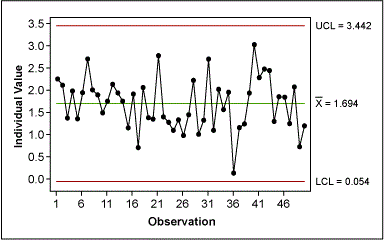Figure 9: Control Chart of Transformed Cycle Time Data

Because the individual values of the transformed data have no practical meaning, the Green Belt had to re-create a control chart for the original data, but this time with the correct control limits (Figure 10). To do this, the Belt used the upper and lower CLs from the control chart of the transformed data and transformed them back into their original values. Because the transformation operation was taking the square root, the back-transformation involved squaring the transformed data:

UCL = UCL’2 = 3.4422 = 11.847
LCL = LCL’2 = -0.0542= 0.003

For the mean, the Belt used the mean of the original data.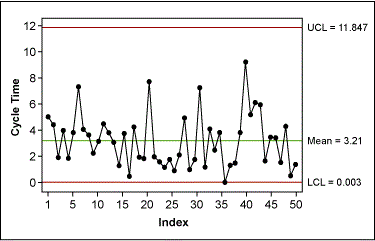Figure 10: Control Chart of the Original Data with Correct Control Limits

This control chart could then be used for the ongoing monitoring of the purchase order-generation process.

##### Arne Buthmann

1.setya

Thanks so much, it’s help me alot

2.Yogesh

Thanks for this amazingly simple explaination of a complex topic.. wonderful

3.Dan

Maybe it’s just because I have read several different explanations of this… and it finally sank in after reading this one.
Great explanation, well done!

Thanks.

4.Dennis

Nice article. For Figure 10, could you explain why the mean was set to the mean of the original data instead of the square of the mean of the transformed data (i.e., 1.694^2)? Also, would the example’s steps change if Box-Cox indicated LN is to be used instead of SQRT? In other words, is it okay to just follow the example if Box-Cox recommends a transformation that really results in a normal distribution regardless of the recommended transformation?

5.Ruben gamez

6.Huchesh H B

Hello sir
which statistical analysis software you have used? please named me..

7.Jessica

Thank you so much for this clear explanation, much clearer than my textbook!

8.Jose

This is explanation is simply the best I have seen. Thank you very much.

9.Hooman

Perfect artilcle . Thank you

10.Hooman

Thanks,it helped me alot, but I have a question for you.After the final calculation,how we can insert these new UCL,LCL and also the Mean in our charts? I couldn’t do it in Minitab.

11.Vibhanshu Bhardwaj

Explanation provided is really simple.

12.Bob

I understand what is being done, I just don’t believe it is useful. I taken non-normal data apply a non-linear transformation to it, and that gives me something I can use?

So why bother?

Because the individual values of the transformed data have no practical meaning, the Green Belt had to re-create a control chart for the original data, but this time with the correct control limits (Figure 10). To do this, the Belt used the upper and lower CLs from the control chart of the transformed data and transformed them back into their original values

13.Mark Meder

Great explaination! Helped me alot!

14.Dr.JOHN MANOHAR

Indeed an useful one.

15.Nizamuddin Siddiqui

Hi,

Can you please tell me when the back-transformation is used the control limits are not matching with the original data control limits but how?

I am waiting for your response.

Thanks

16.Al

Bob,

Its because the belt wants to continue to monitor the process by analysing the data in a control chart. We all know control limits in a control chart assume Normal data. However his data is not normal, as demonstrated. So by carrying out the transformation, and determining the correct limits, these can now be applied to the actual, non-normal, data. Now going forward the only apparent out of control points will be ‘real’ out of control points. This will need to be performed each time data is added to the distribution as this could change the distribution shape, however the process stability can be assessed statistically with this transformation.

Nizamuddin Siddiqui,

Take for example the UCL: in the transformed data UCL is = 3.442. To represent as untransformed you just square it (3.442)^2 = 11.847. This is the reverse of the original transformation for Lambda 0.5

17.Dhiman Banerjee

Thank you for this article. I have seen there is a tendency to convert any non normal data to normal data. Suggest adding situations or rules when transformation is absolutely necessary.

18.Dr Burns

What utter nonsense! Data should NEVER be normalized before control charting. Shewhart charts work for any data distribution as Dr Wheeler has proven.

Please read Dr Wheeler “Normality and the Process Behavior Chart”

19.Brian Patterson

Normal data is not required to complete an individuals control chart. Donald Wheeler has provided statistical proof of this.

20.Scott Hindle

I agree with the previous two gentlemen. I find the first sentence to be mistaken and this is the basis for what follows.
I agree with the author that the lower limit is useless – the boundary conditions puts this at zero. Cycle time can’t be negative. Context has to override the calculated value of -2.7
Interpreting ALL the data in relation to the upper Limit of 9.12, the process is at least approximately, or reasonably, predictable. (Interpreting 50 data in one go is different to interpreting a new value, or a few new values, in a sequential Approach.)
The Green Belt team could then monitor future data after this baseline period using the upper Limit of 9.12.
The explanations of data transformation are easy to read and understand. This clarity, however, doesn’t mean the original data in this case should be transformed. For me, unnecessary and unjustified.

21.Eric

Arne,

Thank you for your article! I thought this was a great example and something I will be able to apply to a project in which I’ve been involved.

Eric

22.Zoltan Minsky

Just because we have tools that can transform data does not mean that we should use those tools to transform data and hide all the signals. I’m sure this article is well-intentioned, but it’s nonsense. Here is a much better treatment of the subject by Donal Wheeler: https://www.qualitydigest.com/inside/quality-insider-column/do-you-have-leptokurtophobia.html

23.Cesar Vasquez

Dear Arne,
What a transformation really does is getting the median of the actual data set become the mean and the median in the new domain where data now closely follows a normal distribution. Therefore, transforming back the value of the mean does not produce a value matching the mean on the original data set but its median.
Although transformations do work in making a non-normal distribution appear normal, at the shop floor level they are of little use. If you don’t believe that, try explaining an operator he should be plotting data and making decision on the square root of seconds or the log of inches…
I do not agree with your statement that “When data is not normally distributed, the cause for non-normality should be determined and appropriate remedial actions should be taken.”
There is nothing wrong with data not following a normal distribution. There is nothing to be fixed. The word “normal” somehow may be misleading to imply that all data must be normal. The term really comes from observation of many processes in nature and manufacturing where the bell-shaped curve naturally or normally occurs.
As you correctly pointed out on the purchase order-generation process example, data are stable, but you would have been able to draw the same conclusion applying non-parametric methods such as a Minitab run-chart that proves the series of original data points to analyze four types of instability. Most likely, all four would come out with high p-Values, indicating a stable process.

24.Israel

You are awesome!! Thank you very much for your practical and usefull information. It help me with my research.